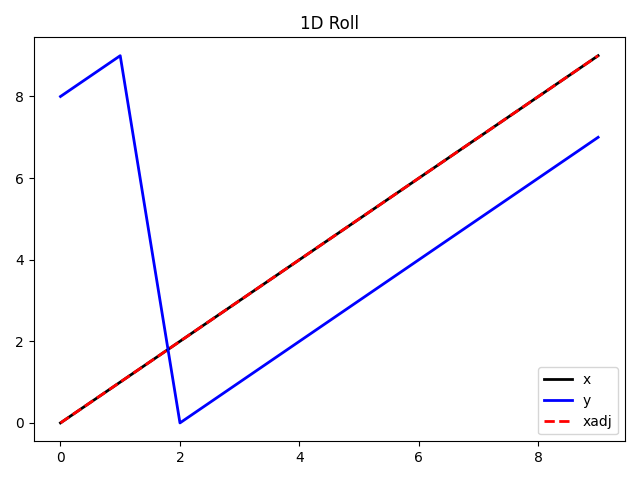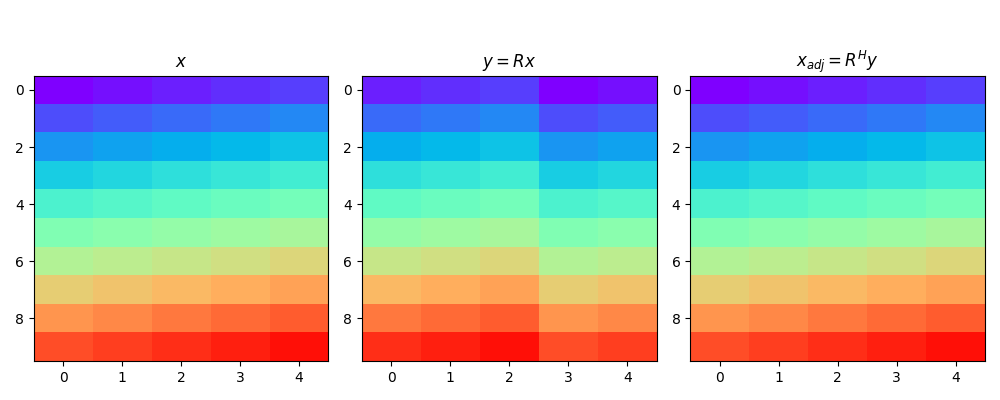# Roll#

This example shows how to use the `pylops.Roll` operator.

This operator simply shifts elements of multi-dimensional array along a specified direction a chosen number of samples.

```import matplotlib.pyplot as plt
import numpy as np

import pylops

plt.close("all")
```

Let’s start with a 1d example. We make a signal, shift it by two samples and then shift it back using its adjoint. We can immediately see how the adjoint of this operator is equivalent to its inverse.

```nx = 10
x = np.arange(nx)

Rop = pylops.Roll(nx, shift=2)

y = Rop * x

plt.figure()
plt.plot(x, "k", lw=2, label="x")
plt.plot(y, "b", lw=2, label="y")
plt.title("1D Roll")
plt.legend()
plt.tight_layout()
```We can now do the same with a 2d array.

```ny, nx = 10, 5
x = np.arange(ny * nx).reshape(ny, nx)

Rop = pylops.Roll(dims=(ny, nx), axis=1, shift=-2)

y = Rop * x

fig, axs = plt.subplots(1, 3, figsize=(10, 4))
fig.suptitle("Roll for 2d data", fontsize=14, fontweight="bold", y=1.15)
axs.imshow(x, cmap="rainbow", vmin=0, vmax=50)
axs.set_title(r"\$x\$")
axs.axis("tight")
axs.imshow(y, cmap="rainbow", vmin=0, vmax=50)
axs.set_title(r"\$y = R x\$")
axs.axis("tight")